## if the midpoint is (2,-14) and the endpoint is (4,-13) what is the other endpoint? solve using the midpoint formula

Question

if the midpoint is (2,-14) and the endpoint is (4,-13) what is the other endpoint? solve using the midpoint formula

in progress 0
5 months 2021-09-05T04:26:09+00:00 1 Answers 3 views 0

## Answers ( )

1. Given:

Coordinates of the midpoint = (2,-14)

Coordinates of one endpoint = (4,-13)

To find:

The coordinates of the another endpoint.

Solution:

Let us assume (a,b) be the another endpoint.

According to the midpoint formula: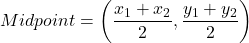By using the midpoint formula, we get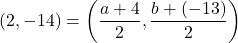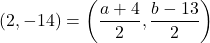On comparing both sides, we get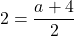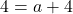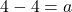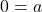And,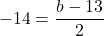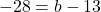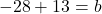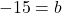Therefore, the another end point is (0,-15).